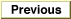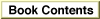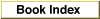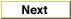# Legacy DocumentImportant: The information in this document is obsolete and should not be used for new development.

Inside Macintosh: QuickDraw GX Environment and Utilities /
Chapter 8 - QuickDraw GX Mathematics / About QuickDraw GX Mathematics

## Number Formats

QuickDraw GX accepts standard integer and floating-point number formats, and defines several fixed-point number formats.

### Integer Formats

Some Quickdraw GX functions and data structures may make use of the standard C language integer formats `short`, `unsigned short`, `long`, and `unsigned long`. The short number format is a 16-bit signed or unsigned integer; the long number format is a 32-bit signed or unsigned integer. Numbers in these formats have the following ranges of values:
FormatRange
short-32, 768 to 32,767
unsigned short0 to 65,535
long-2,147,483,648 to 2,147,483,647
unsigned long0 to 4,294,967,295

### Floating-Point Formats

QuickDraw GX supports conversion to and from the C language single precision floating-point format `float`; double precision floating-point format `double`; and extra precision floating-point format `extended`. QuickDraw GX macros that convert between floating-point numbers and `Fixed` or `fract` numbers can handle all three floating-point formats.

### Fixed-Point Formats

QuickDraw GX defines 16-bit, 32-bit, and 64-bit fixed-point number formats. Fixed-point number formats are integers that are interpreted as real numbers. The conversion between integer number format and a fixed-point number format is described by bias. A bias is a number (commonly expressed as a power of 2) by
which an integer is divided in order to obtain the real number it represents. For
example, the bias for the `Fixed` number format is 16 bits, or 216. In this case, the
integer must be divided by 216 to obtain the real number represented. Therefore, `Fixed` 0x10000 = 65,536/216, or 1.0.

There are one 16-bit, two 32-bit, and one 64-bit number formats:

• The gxColorValue format for fixed-point numbers is a 16-bit unsigned integer. The values range from 0 to 65,535 to represent numbers from 0 to 1. This fixed-point number is described by a bias of 65,535. The integer must be divided by 65,535 to obtain the real number represented. (Its name derives from the fact that it is used to describe color-component values in a Quickdraw GX color structure; see the chapter "Colors and Color-Related Objects" in Inside Macintosh: QuickDraw GX Objects for more information.)
• The `Fixed` format for fixed-point numbers has 16 bits to the left and 16 bits to the right of the binary point. This corresponds to a fixed-point bias of 16 bits. `Fixed` format numbers range from -32,768 to [32,767 + (65,535/65,536)], or approximately 32,768.
• The `fract` format for fixed-point numbers has 2 bits to the left and 30 bits to the right of the binary point. This corresponds to a fixed-point bias of 30 bits. Numbers in `fract` format range from -2 to [2 - (2-30)] or -2 to [1 + (1,073,741,823/1,073,741,824)], or approximately 2.0.
• The `wide` format is a signed integer data type that has 64 bits. It can be given a bias, just as any other integer type can. With a bias of 0 bits, a `wide` format number represents an integer and can range from -9,223,372,036,854,775,808 to 9,223,372,036,854,775,807. With a bias of 16 bits, a `wide` format number represents an extended version of the `Fixed` format (that is, it has the same precision but a larger range) and can range from -140,737,488,355,328 to [140,737,488,355,327 + (65,535/65,536)].

All of the fixed-point number formats except for gxColorValue are two's complement signed integers.

The `wide` data type is defined as a structure that contains an unsigned long integer as its low-order half and a signed long integer as its high-order half. You can convert a `long` into a `wide` in either of two ways:

• First, assign the `long` to the low half of the `wide`. Then, if the `long` is not negative, assign 0 to the high half of the `wide`; if the `long` is negative, assign -1 to the high half of the `wide`.
• Assign the `long` to the high half of the `wide`. Use the `WideShift` function to shift the bits of the `wide` rightward by 32 bits.

The WideShift function is described on page 8-51. The `wide` structure is described on page 8-35.

### Working With Bias in Fixed-Point Operations

`Fixed` numbers have a bias of 16; `fract` numbers have a bias of 30; and `long` and `wide` numbers have a bias of 0. Unless stated otherwise, all biases will be powers of 2. For brevity, we use the convention of describing a bias by the exponent of 2; for example, we say "a bias of 16" instead of "a bias of 16 bits."Operations that are designed to work on a specific number format (such as `FixedMultiply` or `FractDivide` or `WideMultiply`) apply a bias to the result of their operations that reflects the number format they expect. If you understand how the bias is applied, you can use (and even mix) any of several different fixed-point number formats in these functions, and know what bias to use when interpreting the result:

• Operations on `Fixed` numbers (such as `FixedMultiply` and `FixedDivide`) use a bias of 16; operations on `fract` numbers (such as `FractMultiply` and `FractDivide`) use a bias of 30; operations on `long` and `wide` numbers (such as `MultiplyDivide` and `WideDivide`) use a bias of 0.
• When multiplying two fixed-point numbers, the bias of the result is the sum of the biases of the input numbers, minus the bias of the operation. Thus, the result of using `FixedMultiply` to multiply two `Fixed` numbers is a `Fixed` ( = (16 + 16) - 16), as expected. On the other hand, the result of using `FixedMultiply` to multiply a `fract` and a `Fixed` is a `fract` ( = (30 + 16) - 16), and the result of `FractMultiply` on a `fract` and a `Fixed` is a `Fixed` ( = (30 + 16) - 30).
• When dividing two fixed-point numbers, the bias of the result is the operation bias plus the difference between the biases of the input numbers. Thus, as expected, the result of using `FixedDivide` to divide one `Fixed` number by another is a `Fixed` ( = 16 + (16 - 16)). The result of `FixedDivide` on a `fract` divided by a `Fixed` is a `Fract` ( = 16 + (30 - 16)), and the result of `FractDivide` on a `Fixed` divided by a `Fract` is a `Fixed` ( = 30 + (16 - 30)).
• For operations that have no bias, the result is simply the sum or difference of the input biases. For example, if you use `MultiplyDivide` to multiply a `Fixed` by a `fract` and divide the result by a `Fixed`, the result will be a `fract` ( = 16 + 30 - 16). If you use `WideMultiply` to multiply two `Fixed` numbers, the result will have a bias of 32 bits ( = 16 + 16).

Remember also that using the standard C operators + and - to add or subtract fixed-point numbers is meaningful only if the numbers have the same bias. Thus, if you wish to add together a long integer and a `Fixed`, for example, you must first convert one format to the other, or convert both to a common format.

The functions referred to in this section are described in the section "Fixed-Point Operations" beginning on page 8-42, and "Operations on wide Numbers" beginning on page 8-49.